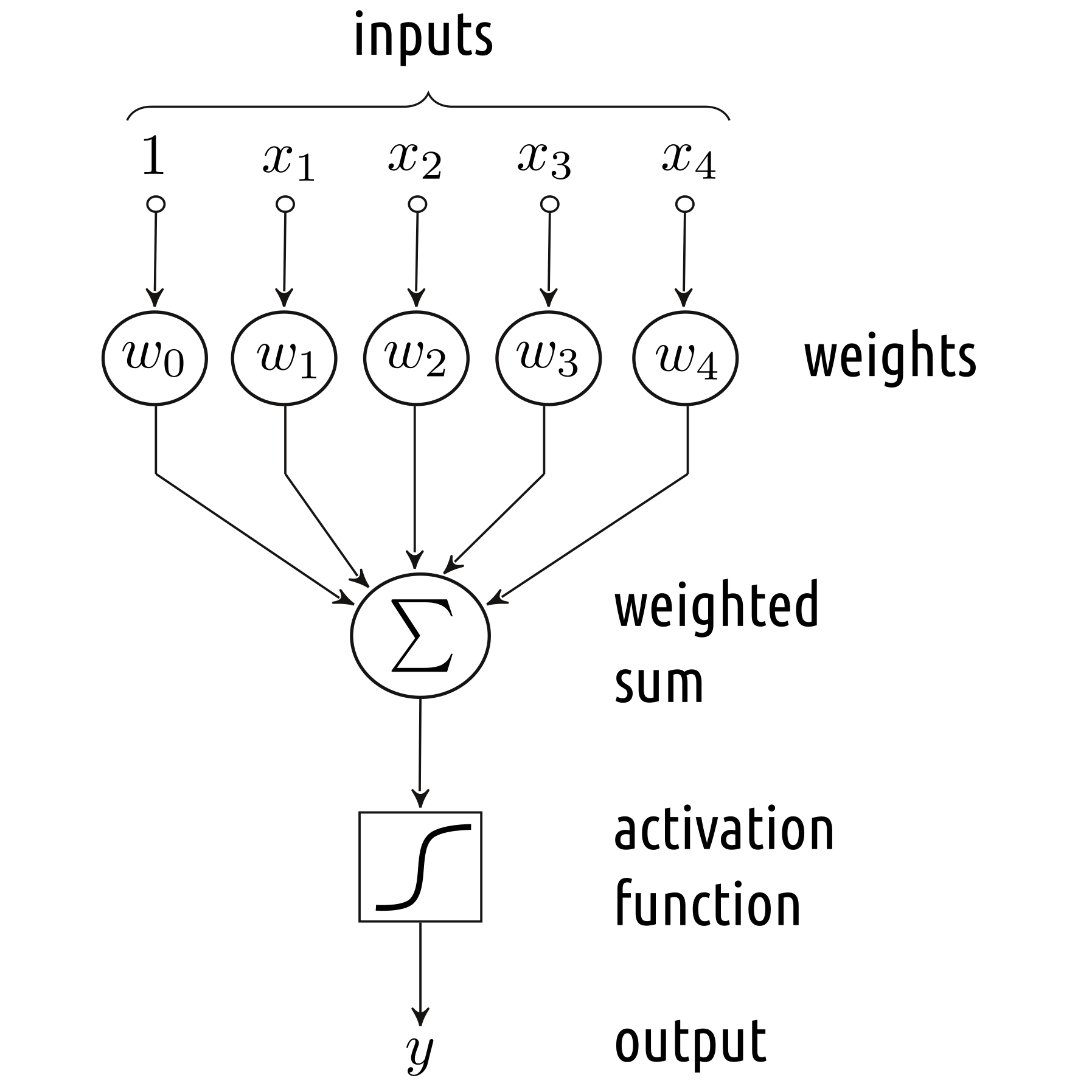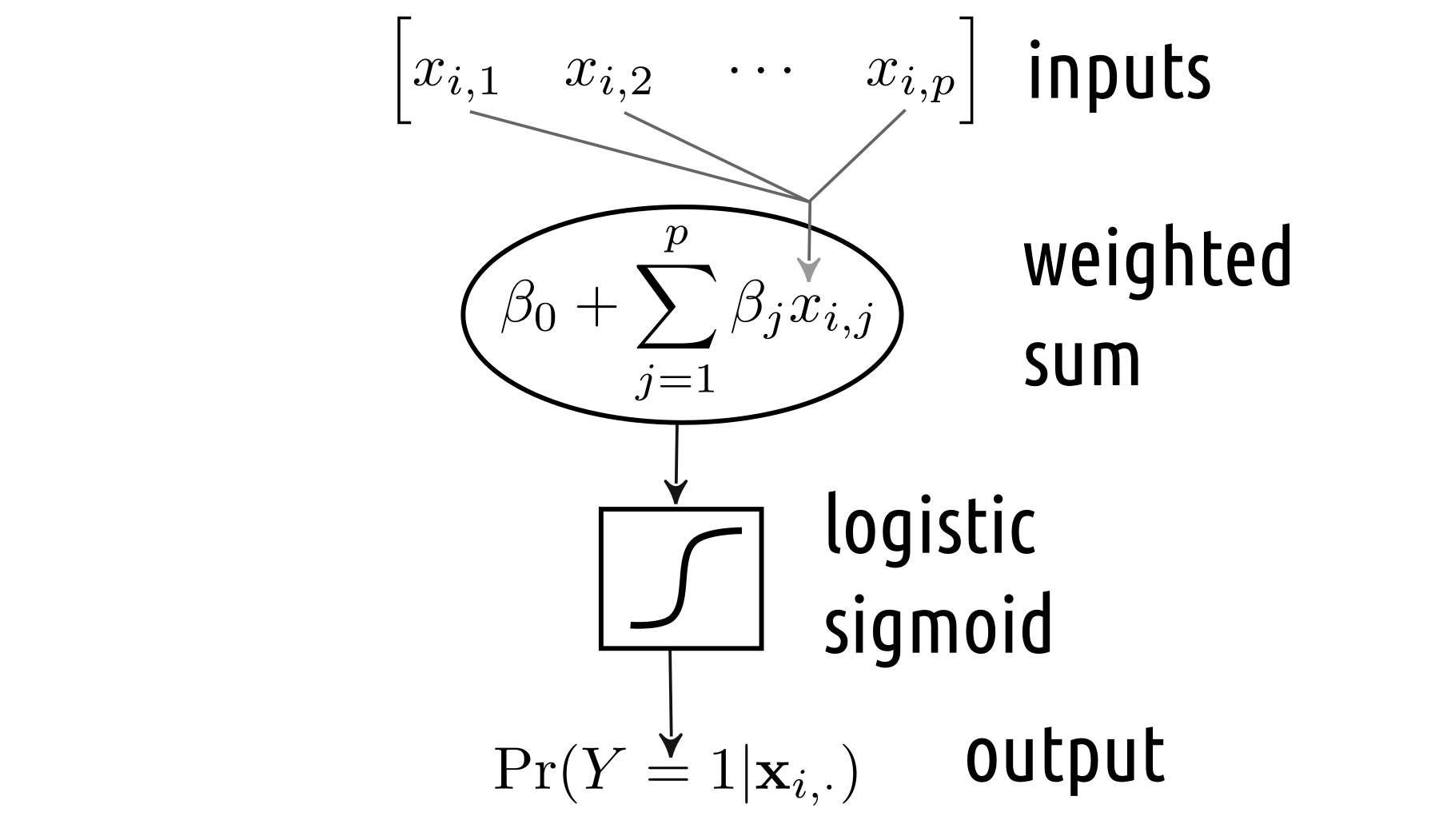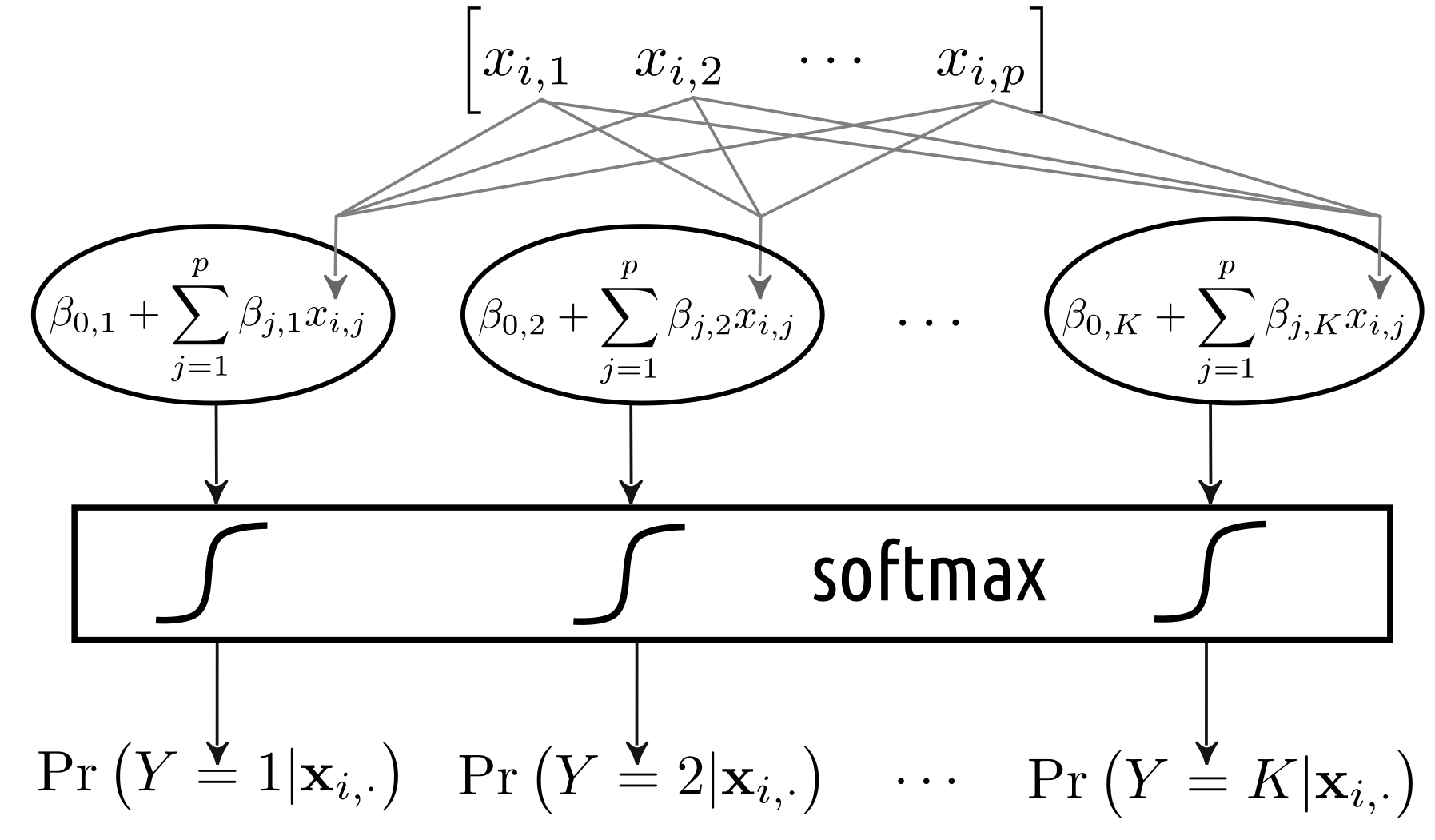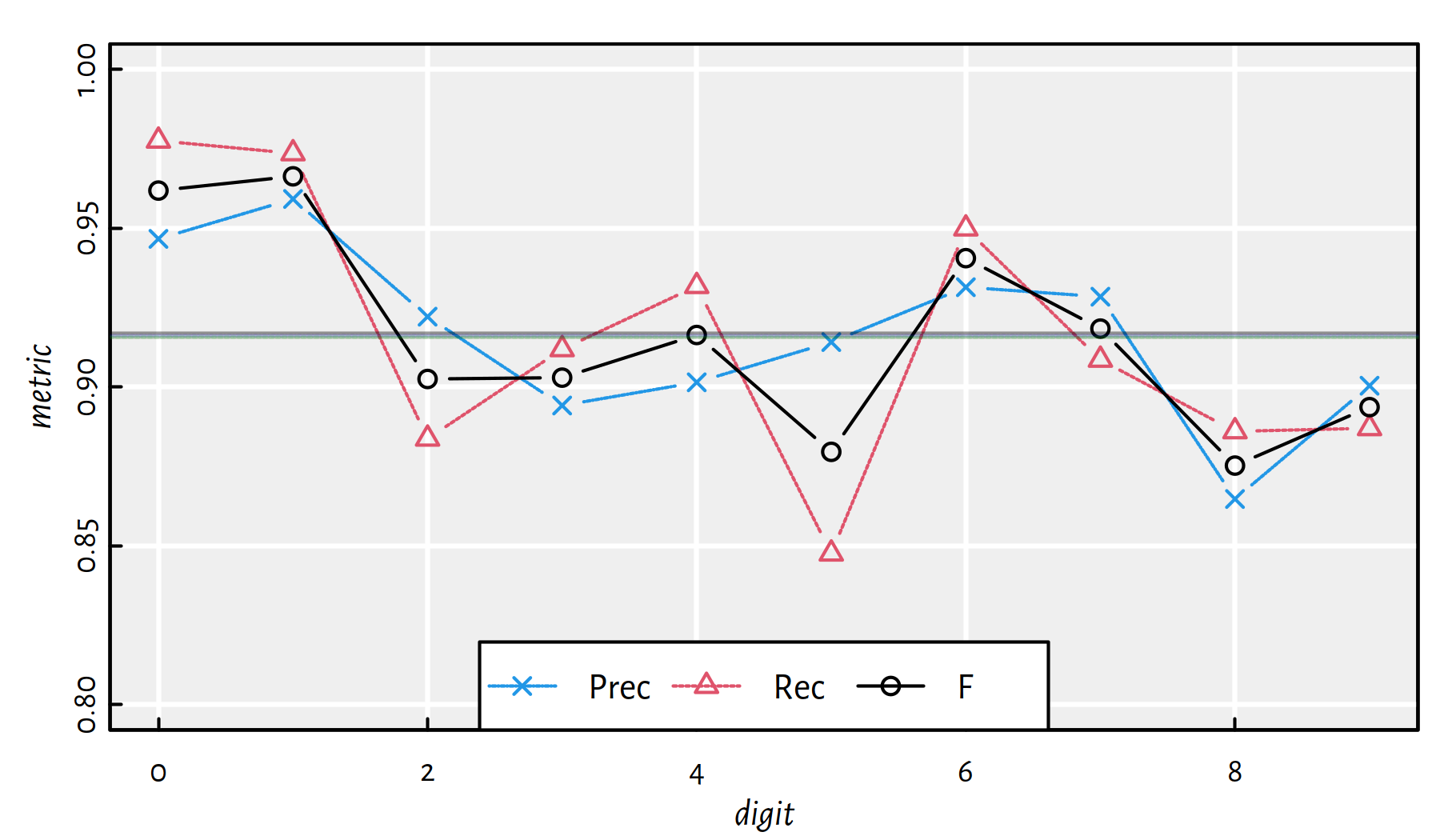## 5.3 Artificial Neural Networks

### 5.3.1 Artificial Neuron

A neuron as a mathematical function:The perceptron (Frank Rosenblatt, 1958) was amongst the first models of artificial neurons:### 5.3.2 Logistic Regression as a Neural Network

The above resembles our binary logistic regression model!

We determine a linear combination (a weighted sum) of 784 inputs and then transform it using the logistic sigmoid “activation” function.A multiclass logistic regression can be depicted as:This is an instance of a:

• single layer (there is only one processing step that consists of 10 units),
• densely connected (all the inputs are connected to all the neurons),
• feed-forward (outputs are generated by processing the inputs directly, there are no loops in the graph etc.)

artificial neural network that uses the softmax as the activation function.

### 5.3.3 Example in R

To train such a neural network (fit a multinomial logistic regression model), we will use the keras package, a wrapper around the state-of-the-art, GPU-enabled TensorFlow library.

# Start with an empty model
model <- keras_model_sequential()
# Add a single layer with 10 units and softmax activation
layer_dense(model, units=10, activation='softmax')
# We will be minimising the cross-entropy,
# sgd == stochastic gradient descent, see the next chapter
compile(model, optimizer='sgd',
loss='categorical_crossentropy')
# Fit the model
fit(model, X_train2, Y_train2, epochs=5)

Predict over the test set and one-hot-decode the output probabilities:

Y_pred2 <- predict(model, X_test2)
round(head(Y_pred2), 2) # predicted class probabilities
##      [,1] [,2] [,3] [,4] [,5] [,6] [,7] [,8] [,9] [,10]
## [1,] 0.00 0.00 0.00 0.00 0.00 0.00 0.00 0.99 0.00  0.00
## [2,] 0.01 0.00 0.85 0.02 0.00 0.02 0.09 0.00 0.01  0.00
## [3,] 0.00 0.95 0.01 0.01 0.00 0.00 0.01 0.01 0.01  0.00
## [4,] 1.00 0.00 0.00 0.00 0.00 0.00 0.00 0.00 0.00  0.00
## [5,] 0.00 0.00 0.01 0.00 0.88 0.00 0.01 0.02 0.01  0.06
## [6,] 0.00 0.97 0.01 0.01 0.00 0.00 0.00 0.01 0.01  0.00
Y_pred <- apply(Y_pred2, 1, which.max)-1 # 1..10 -> 0..9
head(Y_pred, 20) # predicted outputs
##   7 2 1 0 4 1 4 9 6 9 0 6 9 0 1 5 9 7 3 4
head(Y_test, 20) # true outputs
##   7 2 1 0 4 1 4 9 5 9 0 6 9 0 1 5 9 7 3 4

Accuracy on the test set:

mean(Y_test == Y_pred)
##  0.9081

Performance metrics for each digit separately:

i Acc Prec Rec F TN FN FP TP
0 0.9915 0.9365854 0.9795918 0.9576060 8955 20 65 960
1 0.9919 0.9582609 0.9709251 0.9645514 8817 33 48 1102
2 0.9787 0.9191402 0.8701550 0.8939771 8889 134 79 898
3 0.9794 0.8925781 0.9049505 0.8987217 8880 96 110 914
4 0.9813 0.8947368 0.9175153 0.9059829 8912 81 106 901
5 0.9774 0.9193955 0.8183857 0.8659549 9044 162 64 730
6 0.9873 0.9235474 0.9457203 0.9345023 8967 52 75 906
7 0.9812 0.9117647 0.9046693 0.9082031 8882 98 90 930
8 0.9716 0.8429423 0.8706366 0.8565657 8868 126 158 848
9 0.9759 0.8779528 0.8840436 0.8809877 8867 117 124 892

Note how misleading the individual accuracies are! Averages:

##       Acc      Prec       Rec         F
## 0.9816200 0.9076904 0.9066593 0.9067053